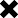### How long should a typical SIP investment last? need help understanding input parameters at this calculator

POSTED BY ON July 22, 2012 11:58 pm ONE COMMENT

Looking at this calculator: http://services.assetmanagement.hsbc.co.in/site/microsites/sip_calculator/sip_calculator.html,

Keeping the following input parameters:
investment: 50,000 (assumed monthly)
5 years
rate of return: 15% (I am assuming this is expected CAGR)

we get a final return as 44.84 lakh while the total investment is 30 lakhs.

I am using the following method to calculate CAGR:
Method 1: (44.84/30)^(1/5) – 1 which is roughly 8.37%
Method 2: 12* ( (44.84/30) ^ (1/60) – 1) which is roughly 8.06%

Are these the right methods to calculate CAGR? I think there must be some mistake somewhere since it’s nowhere close to the input of 15%. I am getting similar inconsistencies with all similar SIP calculators (including this one: http://www.investmentyogi.com/sip-return-calculator.aspx)

Also is 5 years a moderately safe time span for a SIP investment to give a 15-16% CAGR? Is this (either the timespan/CAGR expectance) too optimistic/pessimistic?

## One reply on this article “How long should a typical SIP investment last? need help understanding input parameters at this calculator”

1. Ramesh says:

The difference is because you are comparing putting 50k per month for 5 years versus, putting the lumpsum of all of that amount (30 lakhs) for 5 years.

By normal calculus function, the rate of return will come out to be around half of your presumed return.

I hope you have understood that.

This site uses Akismet to reduce spam. Learn how your comment data is processed.